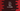# C++ program to print data in a table## Introduction:

In this C++ tutorial, we will learn how to print different data in a table in C++. We will write one program that will print one table using a set of data. With this program, you will learn how to do input/output manipulation in C++.

To print one table, we need to print the columns with equal widths in each row of the table. Also, if the value of a column is less than the size of the column, it should add paddings to the value to make the width equal to other columns. We will use the `iomanip` library with this program.

### setfill() and setw() methods of iomanip:

C++ input/output manipulators library `iomanip` comes with a lot of useful methods. In this example, we are going to use the `setfill()` and `setw()` methods.

The `setw()` method is used to change the width of the output field. The syntax of the `setw` method is:

``setw(int n)``

Where the parameter `n` is the filled width. For example, if we passed the parameter `n` as 4 and if we try to print the word ‘one’, which is a three-letter word, the output will add one blank space to the output to make it equal to the width 4.

The `setfill` method takes one character as the argument and uses it as the fill character. The syntax of the `setfill` method is:

``setfill (char_type c);``

Let’s consider the following example:

``````#include <iostream>
#include <iomanip>

using namespace std;

int main()
{
cout
<< setfill('*')
<< setw(5)
<< "one" << endl;

return 0;
}``````

It will print:

``**one``

The width is set as 5 and the fill character is *. The `cout` syntax is printing the word “one” which is a string of three characters. Since the width is 5, it will add two fill characters to the start of the string.

### std::left and std::right:

The `std::left` and `std::right` are used to change the position of the fill characters of the output stream. The fill characters are added to the left of a string by default. We can use `std::left` to print the fill characters to the end. For example,

``````#include <iostream>
#include <iomanip>

using namespace std;

int main()
{
cout
<< setfill('*')
<< left
<< setw(5)
<< "one" << endl;

return 0;
}``````

This is similar to the previous example. We are using `std::left` to add the fill characters to the end. If you run this program, it will print:

``one**``

### C++ program to print data table with object class:

Let’s take a look at the below program:

``````#include <iostream>
#include <iomanip>

using namespace std;

class Student
{
public:
string studentName;
int studentAge;
int studentMarks;

Student(string name, int age, int marks, int year)
{
studentName = name;
studentAge = age;
studentMarks = marks;
}
};

int main()
{
Student studentArray = {Student("Alex", 20, 80, 2018), Student("Bob", 21, 82, 2018), Student("Chandler", 23, 85, 2017), Student("Rose", 18, 89, 2019)};

cout
<< left
<< setw(10)
<< "Name"
<< left
<< setw(5)
<< "Age"
<< left
<< setw(8)
<< "Marks"
<< left
<< setw(5)
<< "Year"
<< endl;

for (int i = 0; i < 4; i++)
{
cout
<< left
<< setw(10)
<< studentArray[i].studentName
<< left
<< setw(5)
<< studentArray[i].studentAge
<< left
<< setw(8)
<< studentArray[i].studentMarks
<< left
<< setw(5)
<< endl;
}
return 0;
}``````

• The `Student` class is used to store the info of a student. We can assign the properties to an object with the constructor of this class.
• We have set different widths for each column. The first column width is 10, the second column width is 5, the third column width is 8, and the last column width is 5.
• The `for` loop is printing the values of the `studentArray` array. Before a value is printed, we are assigning the width with the `setw` method and `std::left` will add the fill characters to the end. Since we haven’t defined any fill characters, it will use blank spaces by default.

It will print the below output:

``````Name      Age  Marks   Year
Alex      20   80      2018
Bob       21   82      2018
Chandler  23   85      2017
Rose      18   89      2019``````

The width is important. If it is less than the size of its content, the content will overflow.

For example:

``````#include <iostream>
#include <iomanip>

using namespace std;

int main()
{
cout
<< left
<< setw(1)
<< "one" << endl;

return 0;
}``````

It will print the string “one” even though we set the width as 1.